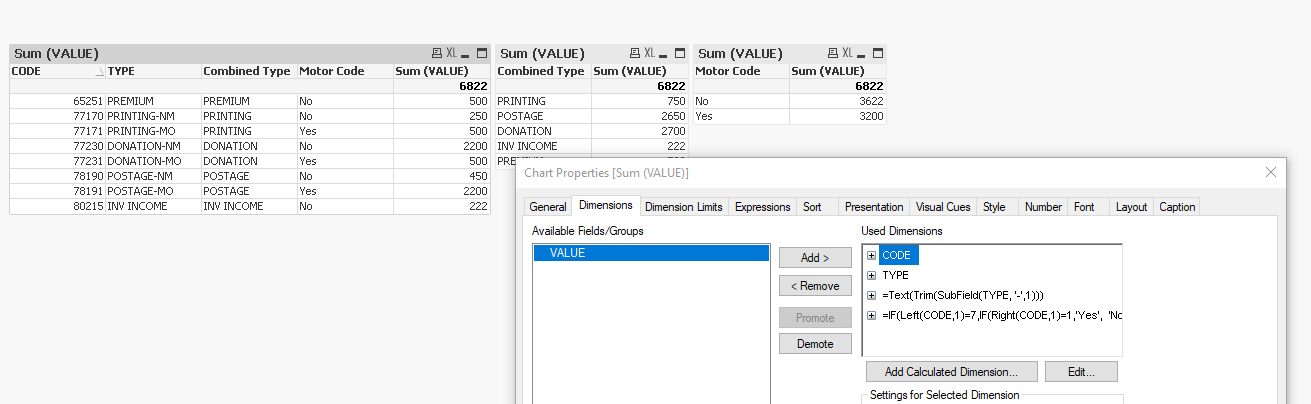New to QlikView

Discussion board where members can get started with QlikView.

Announcements
QlikWorld 2020: Join us May 11 - 14, 2020 in Phoenix, AZ. Register early and save \$400. Learn More
Valued Contributor

Combining codes together & present the value adding both MO & NM

Dear Friends

If I am to combine both codes define for MO & NM together & to present value adding both, how it is done both at script level & front end. Also if the serial of the codes end with 1 such as 171,191 etc, I need to give a function to pick all codes ends with 1 as Motor Codes. Also this should be only from  seventy code series such as 77151,

1 Solution

Accepted Solutions
Valued Contributor

Re: Combining codes together & present the value adding both MO & NM

Script:

*,
SubField(TYPE, '-',1) as Combined_Type,
IF(Left(CODE,1)=7, IF(Right(CODE,1)=1, 'Yes','No'),'No') as Motor_Code;
LOAD TYPE, CODE, VALUE
FROM (ooxml, embedded labels, table is Sheet1);

UI:Combined Type Dimension: Text(Trim(SubField(TYPE, '-',1)))

Motor Code Dimension: IF(Left(CODE,1)=7,IF(Right(CODE,1)=1,'Yes', 'No'),'No')

You can create the same dimensions as columns in the script.

6 Replies
Valued Contributor

Re: Combining codes together & present the value adding both MO & NM

Refer the attachment

Valued Contributor

Re: Combining codes together & present the value adding both MO & NM

Please find the attached and let me know if it matches your requirement.

Valued Contributor

Re: Combining codes together & present the value adding both MO & NM

Created two more charts for visualizations.

PFA.

Valued Contributor

Re: Combining codes together & present the value adding both MO & NM

Hi Vamsee

Sorry for the delay in response as I was away from home. Could you please send me your working typed in the body of the reply the fact is that I cannot open your answer. I am using a personnel edition of qlik view.

Thanks

Neville

Valued Contributor

Re: Combining codes together & present the value adding both MO & NM

Script:

*,
SubField(TYPE, '-',1) as Combined_Type,
IF(Left(CODE,1)=7, IF(Right(CODE,1)=1, 'Yes','No'),'No') as Motor_Code;
LOAD TYPE, CODE, VALUE
FROM (ooxml, embedded labels, table is Sheet1);

UI:Combined Type Dimension: Text(Trim(SubField(TYPE, '-',1)))

Motor Code Dimension: IF(Left(CODE,1)=7,IF(Right(CODE,1)=1,'Yes', 'No'),'No')

You can create the same dimensions as columns in the script.

Valued Contributor

Re: Combining codes together & present the value adding both MO & NM

Thanks Vamsee,

This is what I needed

Neville